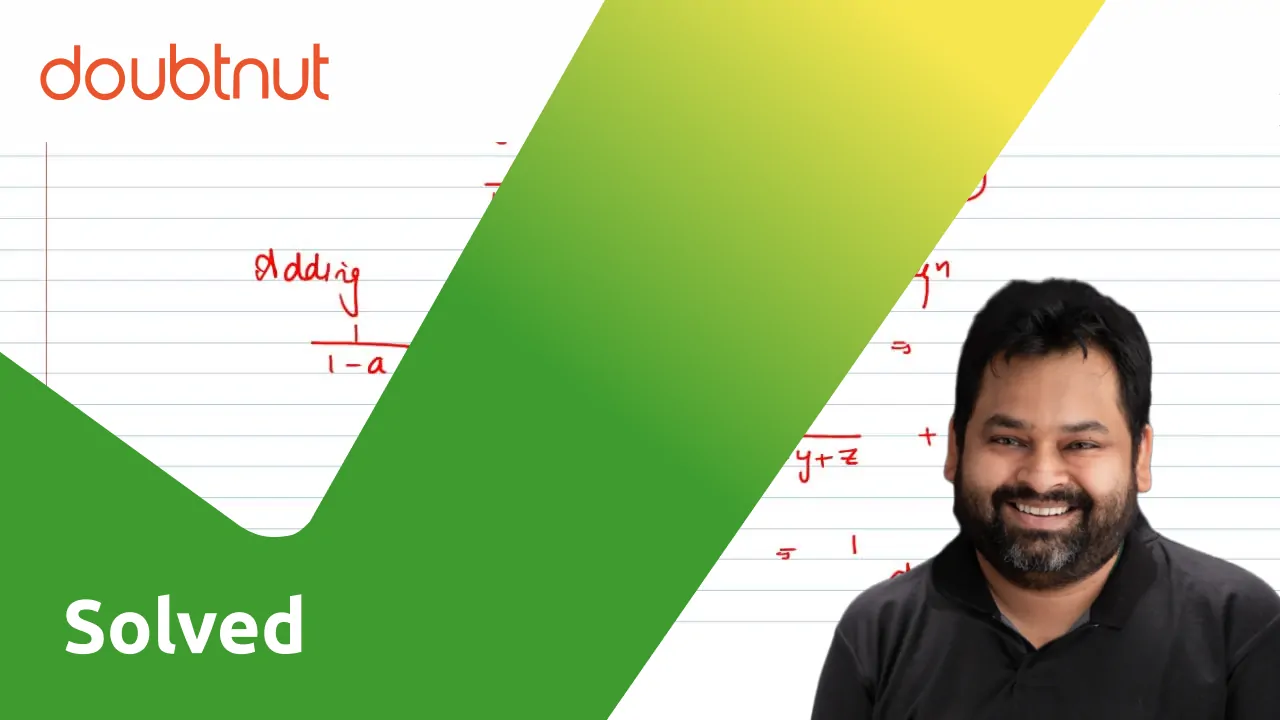Doubtnut App - Get 50 Lakh+ video solutions better than GoogleInstall Now

# if the system of equation ax+y+z=0,x+by=z=0,andx+y+cz=0(a,b,c≠1) has a nontrival solution , then the value of 11−a+11−b+11−cis:

Video Solution